# 8.5 Simplify complex rational expressions

 Page 1 / 3
By the end of this section, you will be able to:
• Simplify a complex rational expression by writing it as division
• Simplify a complex rational expression by using the LCD

Before you get started, take this readiness quiz.

If you miss a problem, go back to the section listed and review the material.

1. Simplify: $\frac{\frac{3}{5}}{\frac{9}{10}}.$
If you missed this problem, review [link] .
2. Simplify: $\frac{1-\frac{1}{3}}{{4}^{2}+4·5}.$
If you missed this problem, review [link] .

Complex fractions are fractions in which the numerator or denominator contains a fraction. In Chapter 1 we simplified complex fractions like these:

$\frac{\frac{3}{4}}{\frac{5}{8}}\phantom{\rule{4em}{0ex}}\frac{\frac{x}{2}}{\frac{xy}{6}}$

In this section we will simplify complex rational expressions , which are rational expressions with rational expressions in the numerator or denominator.

## Complex rational expression

A complex rational expression    is a rational expression in which the numerator or denominator contains a rational expression.

Here are a few complex rational expressions:

$\frac{\frac{4}{y-3}}{\frac{8}{{y}^{2}-9}}\phantom{\rule{7em}{0ex}}\frac{\frac{1}{x}+\frac{1}{y}}{\frac{x}{y}-\frac{y}{x}}\phantom{\rule{7em}{0ex}}\frac{\frac{2}{x+6}}{\frac{4}{x-6}-\frac{4}{{x}^{2}-36}}$

Remember, we always exclude values that would make any denominator zero.

We will use two methods to simplify complex rational expressions.

## Simplify a complex rational expression by writing it as division

We have already seen this complex rational expression earlier in this chapter.

$\frac{\frac{6{x}^{2}-7x+2}{4x-8}}{\frac{2{x}^{2}-8x+3}{{x}^{2}-5x+6}}$

We noted that fraction bars tell us to divide, so rewrote it as the division problem

$\left(\frac{6{x}^{2}-7x+2}{4x-8}\right)÷\left(\frac{2{x}^{2}-8x+3}{{x}^{2}-5x+6}\right)$

Then we multiplied the first rational expression by the reciprocal of the second, just like we do when we divide two fractions.

This is one method to simplify rational expressions. We write it as if we were dividing two fractions.

Simplify: $\frac{\frac{4}{y-3}}{\frac{8}{{y}^{2}-9}}.$

## Solution

$\begin{array}{cccc}& & & \hfill \phantom{\rule{5em}{0ex}}\frac{\frac{4}{y-3}}{\frac{8}{{y}^{2}-9}}\hfill \\ \\ \\ \text{Rewrite the complex fraction as division.}\hfill & & & \hfill \phantom{\rule{5em}{0ex}}\frac{4}{y-3}÷\frac{8}{{y}^{2}-9}\hfill \\ \\ \\ \begin{array}{c}\text{Rewrite as the product of first times the}\hfill \\ \text{reciprocal of the second.}\hfill \end{array}\hfill & & & \hfill \phantom{\rule{5em}{0ex}}\frac{4}{y-3}·\frac{{y}^{2}-9}{8}\hfill \\ \\ \\ \text{Multiply.}\hfill & & & \hfill \phantom{\rule{5em}{0ex}}\frac{4\left({y}^{2}-9\right)}{8\left(y-3\right)}\hfill \\ \\ \\ \text{Factor to look for common factors.}\hfill & & & \hfill \phantom{\rule{5em}{0ex}}\frac{4\left(y-3\right)\left(y+3\right)}{4·2\left(y-3\right)}\hfill \\ \\ \\ \text{Remove common factors.}\hfill & & & \hfill \phantom{\rule{5em}{0ex}}\frac{\overline{)4}\overline{)\left(y-3\right)}\left(y+3\right)}{\overline{)4}·2\overline{)\left(y-3\right)}}\hfill \\ \\ \\ \text{Simplify.}\hfill & & & \hfill \phantom{\rule{5em}{0ex}}\frac{y+3}{2}\hfill \end{array}$

Are there any value(s) of $y$ that should not be allowed? The simplified rational expression has just a constant in the denominator. But the original complex rational expression    had denominators of $y-3$ and ${y}^{2}-9$ . This expression would be undefined if $y=3$ or $y=-3$ .

Simplify: $\frac{\frac{2}{{x}^{2}-1}}{\frac{3}{x+1}}.$

$\frac{2}{3\left(x-1\right)}$

Simplify: $\frac{\frac{1}{{x}^{2}-7x+12}}{\frac{2}{x-4}}.$

$\frac{1}{2\left(x-3\right)}$

Fraction bars act as grouping symbols. So to follow the Order of Operations, we simplify the numerator and denominator as much as possible before we can do the division.

Simplify: $\frac{\frac{1}{3}+\frac{1}{6}}{\frac{1}{2}-\frac{1}{3}}.$

## Solution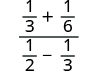Simplify the numerator and denominator. Find the LCD and add the fractions in the numerator. Find the LCD and add the fractions in the denominator.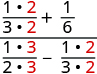Simplify the numerator and denominator.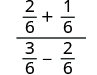Simplify the numerator and denominator, again.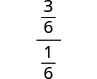Rewrite the complex rational expression as a division problem.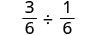Multiply the first times by the reciprocal of the second.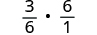Simplify.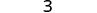Simplify: $\frac{\frac{1}{2}+\frac{2}{3}}{\frac{5}{6}+\frac{1}{12}}.$

$\frac{14}{11}$

Simplify: $\frac{\frac{3}{4}-\frac{1}{3}}{\frac{1}{8}+\frac{5}{6}}.$

$\frac{10}{23}$

## How to simplify a complex rational expression by writing it as division

Simplify: $\frac{\frac{1}{x}+\frac{1}{y}}{\frac{x}{y}-\frac{y}{x}}.$

## Solution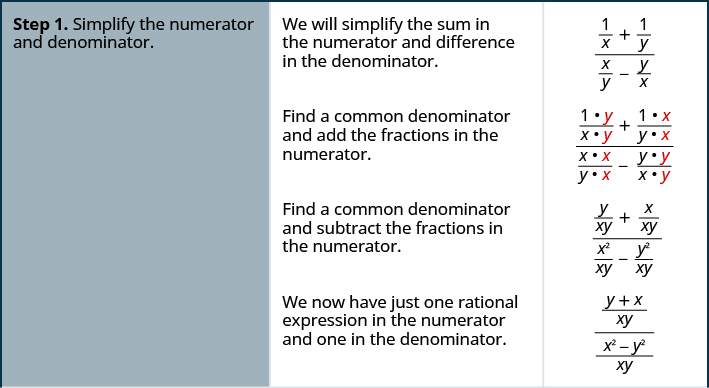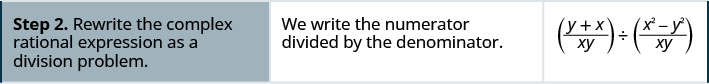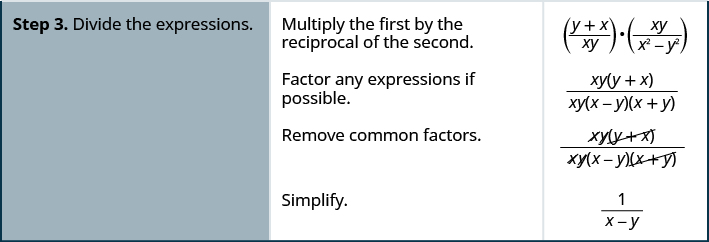Simplify: $\frac{\frac{1}{x}+\frac{1}{y}}{\frac{1}{x}-\frac{1}{y}}.$

$\frac{y+x}{y-x}$

Simplify: $\frac{\frac{1}{a}+\frac{1}{b}}{\frac{1}{{a}^{2}}-\frac{1}{{b}^{2}}}.$

$\frac{ab}{b-a}$

## Simplify a complex rational expression by writing it as division.

1. Simplify the numerator and denominator.
2. Rewrite the complex rational expression as a division problem.
3. Divide the expressions.

Aziza is solving this equation-2(1+x)=4x+10
No. 3^32 -1 has exactly two divisors greater than 75 and less than 85 what is their product?
x^2+7x-19=0 has Two solutions A and B give your answer to 3 decimal places
3. When Jenna spent 10 minutes on the elliptical trainer and then did circuit training for20 minutes, her fitness app says she burned 278 calories. When she spent 20 minutes onthe elliptical trainer and 30 minutes circuit training she burned 473 calories. How manycalories does she burn for each minute on the elliptical trainer? How many calories doesshe burn for each minute of circuit training?
.473
Angelita
?
Angelita
John left his house in Irvine at 8:35 am to drive to a meeting in Los Angeles, 45 miles away. He arrived at the meeting at 9:50. At 3:30 pm, he left the meeting and drove home. He arrived home at 5:18.
p-2/3=5/6 how do I solve it with explanation pls
P=3/2
Vanarith
1/2p2-2/3p=5p/6
James
Cindy
4.5
Ruth
is y=7/5 a solution of 5y+3=10y-4
yes
James
Cindy
Lucinda has a pocketful of dimes and quarters with a value of $6.20. The number of dimes is 18 more than 3 times the number of quarters. How many dimes and how many quarters does Lucinda have? Rhonda Reply Find an equation for the line that passes through the point P ( 0 , − 4 ) and has a slope 8/9 . Gabriel Reply is that a negative 4 or positive 4? Felix y = mx + b Felix if negative -4, then -4=8/9(0) + b Felix -4=b Felix if positive 4, then 4=b Felix then plug in y=8/9x - 4 or y=8/9x+4 Felix Macario is making 12 pounds of nut mixture with macadamia nuts and almonds. macadamia nuts cost$9 per pound and almonds cost $5.25 per pound. how many pounds of macadamia nuts and how many pounds of almonds should macario use for the mixture to cost$6.50 per pound to make?
Nga and Lauren bought a chest at a flea market for $50. They re-finished it and then added a 350 % mark - up Makaila Reply$1750
Cindy
the sum of two Numbers is 19 and their difference is 15
2, 17
Jose
interesting
saw
4,2
Cindy
Felecia left her home to visit her daughter, driving 45mph. Her husband waited for the dog sitter to arrive and left home 20 minutes, or 13 hour later. He drove 55mph to catch up to Felecia. How long before he reaches her?
hola saben como aser un valor de la expresión
NAILEA
integer greater than 2 and less than 12
2 < x < 12
Felix
I'm guessing you are doing inequalities...
Felix
Actually, translating words into algebraic expressions / equations...
Felix
hi
Darianna
hello
Mister
Eric here
Eric
6
Cindy

#### Get Jobilize Job Search Mobile App in your pocket Now!By OpenStaxBy Brooke DelaneyBy Michael SagBy Ann SchlosserBy OpenStaxBy OpenStaxByBy Madison ChristianBy OpenStaxBy Sandhills MLT